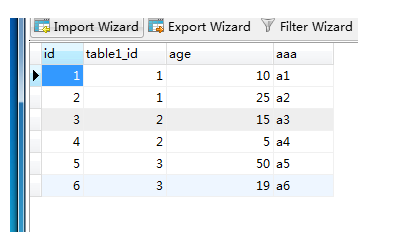# MySql按一个字段分组并且取另一个字段最大值的记录select * from (select * from table2 order by age desc) as a group by a.table1_id

select a.* from table2 as a where age = (select max(age) from table2 where a.table1_id=table1_id)

select a.* from table2 as a where not exists (select * from table2 where table1_id=a.table1_id and age>a.age)

select a.* from table2 as a where exists (select count(*) from table2 where table1_id=a.table1_id and age>a.age having count(*)=0)